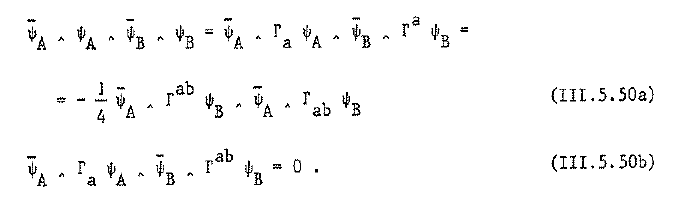# nLab D=5 supergravity

Contents

### Context

#### Gravity

gravity, supergravity

# Contents

## Idea

supergravity in dimension 5. For $N = 1$ this arises from 11-dimensional supergravity by KK-compactification on a Calabi-Yau manifold of complex dimension 3 (see at M-theory on Calabi-Yau manifolds), hence serves as the low-energy effective field theory of the strong-coupling version of Calabi-Yau compactifications of type IIA string theory (see supersymmetry and Calabi-Yau manifolds)

$\array{ 11d \; SuGra\; on\; S^1 \times Y_6 \times X_4 &\longrightarrow& 5d \; SuGra\; on\; S^1 \times X_4 && strong \; coupling \\ \downarrow && \downarrow \\ 10d\; tpye \;IIA\; Sugra\; on \; Y_6 \times X_4 &\longrightarrow& 10d\; Sugra\; on \; X_4 && weak \; coupling }$

## Properties

### 5d Chern-Simons term

This theory has a 2-form field strength $F_2$, locally $F_2 = d A$, with a 5d Chern-Simons theory action functional locally of the form $\propto \int_X F_2 \wedge F_2 \wedge A$ (e.g. Castellani-D’Auria-Fre (III.5.70), Gauntlet-Myers-Townsend 98, p. 3, GGHPR 02 (2.1), Bonetti-Grimm-Hohenegger 13). Hence its equation of motion is of the non-linear form

$d F_3 = F_2 \wedge F_2$

with $F_3 \coloneqq \star F_2$ the Hodge dual of $F_2$ (GGHPR 02 (2.2)).

This is reflected in the corresponding cochains on super Minkowski spacetime

$\mu_{D0,5d} = \overline{\psi}_A \psi_A \phantom{AAA} \mu_{string,5d} = \overline{\psi}_A\Gamma_a \psi_A \wedge e^a$

satisfying

$d \mu_{string,5d} = \mu_{D0,5d} \wedge \mu_{D0,5d} \,.$(the other Fierz identity (III.5.50a) gives the cocycle for the membrane (super 2-brane in 5d) $\mu_{membrane,5d} \coloneqq \frac{i}{2}\overline{\psi}_A \Gamma_{a b} \psi \wedge e^a \wedge e^b$, $d \mu_{membrane,5d} = 0$, that appears already in the old brane scan. )

This is a lower dimensional analogue to the situation for the C-field $G_4$ (locally $G_4 = d C$) in 11-dimensional supergravity, which has a Chern-Simons term locally of the form $\propto \int G_4 \wedge G_4 \wedge C$ and hence the equation of motion

$d G_7 \;=\; -\tfrac{1}{2}G_4 \wedge G_4$

with $G_7 = \star G_4$.

### Black holes and black rings

The first black ring solution in 5d sugra was found in (Elvang-Emparan-Mateos-Reall 04, Elvang-Emparan-Mateos-Reall 05).

Supersymmetric black holes exist precisely only in dimensions 4 and 5 (Gauntlett-Myers-Townsend 98). These play a key role in the discussion of black holes in string theory.

(There are supersymmetric particle-like solutions of $d \gt 5$ supergravity theories that are sometimes called black holes, but these are always singular. There are also supersymmetric black holes in $d = 3$, but the spacetime in that case is asymptotically anti-de Sitter spacetime rather than asymptotically flat. Of course, there are non-singular supersymmetric black brane solutions in various $d \geq 4$ supergravity theories but these are neither ‘particle-like’ nor, strictly speaking, asymptotically flat.)

### Via Calabi-Yau compactification of 11d supergravity

Discussion of 5d supergravity as a KK-compactification of 11-dimensional supergravity on a Calabi-Yau manifold of complex dimension 3 (M-theory on Calabi-Yau manifolds) is discussed in

### U-duality

supergravity gauge group (split real form)T-duality group (via toroidal KK-compactification)U-dualitymaximal gauged supergravity
$SL(2,\mathbb{R})$1$SL(2,\mathbb{Z})$ S-duality10d type IIB supergravity
SL$(2,\mathbb{R}) \times$ O(1,1)$\mathbb{Z}_2$$SL(2,\mathbb{Z}) \times \mathbb{Z}_2$9d supergravity
SU(3)$\times$ SU(2)SL$(3,\mathbb{R}) \times SL(2,\mathbb{R})$$O(2,2;\mathbb{Z})$$SL(3,\mathbb{Z})\times SL(2,\mathbb{Z})$8d supergravity
SU(5)$SL(5,\mathbb{R})$$O(3,3;\mathbb{Z})$$SL(5,\mathbb{Z})$7d supergravity
Spin(10)$Spin(5,5)$$O(4,4;\mathbb{Z})$$O(5,5,\mathbb{Z})$6d supergravity
E6$E_{6(6)}$$O(5,5;\mathbb{Z})$$E_{6(6)}(\mathbb{Z})$5d supergravity
E7$E_{7(7)}$$O(6,6;\mathbb{Z})$$E_{7(7)}(\mathbb{Z})$4d supergravity
E8$E_{8(8)}$$O(7,7;\mathbb{Z})$$E_{8(8)}(\mathbb{Z})$3d supergravity
E9$E_{9(9)}$$O(8,8;\mathbb{Z})$$E_{9(9)}(\mathbb{Z})$2d supergravityE8-equivariant elliptic cohomology
E10$E_{10(10)}$$O(9,9;\mathbb{Z})$$E_{10(10)}(\mathbb{Z})$
E11$E_{11(11)}$$O(10,10;\mathbb{Z})$$E_{11(11)}(\mathbb{Z})$

### $N = 2$ supersymmetry

Consider super Lie algebra cocoycles on $N =2$ 5d super-Minkowski spacetime (as in the brane scan).

With the notation as used at super Minkowski spacetime – Canonical coordinates, there are now two copies of spinor-valued 1-forms, denoted $\psi_1$ and $\psi_2$. We use indices of the form $A,B, \cdots$ for these. Then the non-trivial bit of the Chevalley-Eilenberg algebra differential for $N = 2$, $d = 5$ super Minkowski spacetime is

$d_{CE} e^a = - \tfrac{i}{2} \overline{\psi}_A \wedge \Gamma^a \psi_A$

where summation over repeated indices is understood.

There is a Fierz identity

$\overline{\psi}_A \wedge \psi_A \wedge \overline{\psi}_B \wedge \psi_B \;=\; \overline{\psi}_A \wedge \Gamma_a \psi_A \wedge \overline{\psi}_B \wedge \Gamma^a \psi_B \,.$

This implies that

$d_{CE} (\overline{\psi}_A \Gamma^a \psi_A \wedge e_a) \;\propto\; (\overline{\psi}_A \wedge \Gamma^a \psi_A) \wedge (\overline{\psi}_B \wedge \Gamma^a \psi_B) \,.$

There is a 4-cocycle of the form

$\mu_2 = \epsilon^{A B} \overline{\psi}_A \wedge \Gamma^{a b} \psi_B \wedge e_a \wedge e_b \,.$

Construction of 5d gauged supergravity via D'Auria-Fré formulation of supergravity is in

surveyed in

### General

General discussion includes

### Via M-theory on Calabi-Yau 3-folds

Discussion via KK-compactification as M-theory on Calabi-Yau manifolds includes

Further discussion of the 5d Chern-Simons term includes

(one-loop corrections).

### Gauged sugra

The maximal 5d gauged supergravity was first constructed in

See (ACFG 01).

• Murat Gunaydin, Marco Zagermann, The Gauging of Five-dimensional, $N=2$ Maxwell-Einstein Supergravity Theories coupled to Tensor Multiplets, Nucl.Phys.B572:131-150,2000 (arXiv:hep-th/9912027)

• Murat Gunaydin, Marco Zagermann, The Vacua of 5d, $N=2$ Gauged Yang-Mills/Einstein/Tensor Supergravity: Abelian Case, Phys.Rev.D62:044028,2000 (arXiv:hep-th/0002228)

• A. Ceresole, Gianguido Dall'Agata, General matter coupled $N=2$, $D=5$ gauged supergravity, Nucl.Phys. B585 (2000) 143-170 (arXiv:hep-th/0004111)

• John Ellis, Murat Gunaydin, Marco Zagermann, Options for Gauge Groups in Five-Dimensional Supergravity, JHEP 0111:024,2001 (arXiv:hep-th/0108094)

### Horava-Witten compactification

Discussion of KK-compactification on $S^1/(\mathbb{Z}/2)$-orbifolds (the version of Horava-Witten theory after dimensional reduction) is discussed in

• Filipe Paccetti Correia, Michael G. Schmidt, Zurab Tavartkiladze, 4D Superfield Reduction of 5D Orbifold SUGRA and Heterotic M-theory (arXiv:hep-th/0602173)

### Black hole solutions

Discussion of lifts of 4d black holes to 5d black holes and black rings and embedding as black holes in string theory includes

Review:

• Per Kraus, Lectures on black holes and the $AdS_3$/$CFT_2$ correspondence (arXiv:hep-th/0609074)

Stringy black holes in five dimensions, 2007 (pdf slides)

Further defect branes: